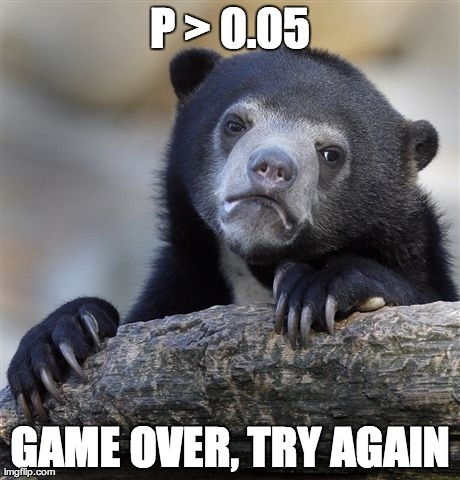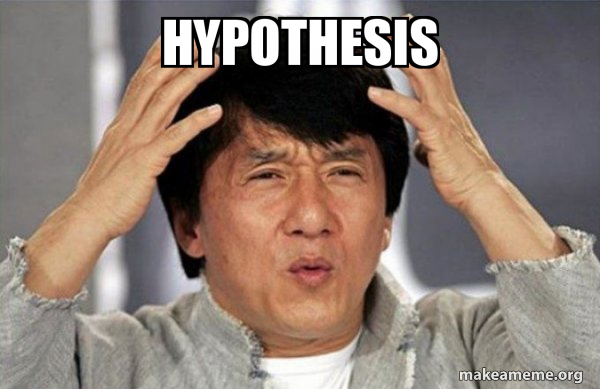# Hyothesis

Published:

This post covers Hypothesis Testing.

# Hypothesis Testing

Dependent Variable
(Measured in scale from 1 to 10)
Sample Mean $\bar{X}$
(n=20)
ProbabilityLikely or Unlikely
Student Engagement$\bar{X}_E =$ Something$p \sim 0.05$
Student Learning$\bar{X}_L =$ Something$p \sim 0.10$
• Threshold is difficult to decide - likely or unlikely

|||| | ———————————– | ———————————————————— | ———————————————————— |

# $\alpha$ Levels of Likelihood (unlikelihood) - One Tailed• If the probability of getting a sample mean is less than
• $\alpha = 0.05 (5\%)$
• $\alpha = 0.01 (1\%)$
• $\alpha = 0.001 (0.1\%)$
• then it is considered unlikely.
• If the probability of getting a particular sample mean is less than $\alpha$ (0.05, 0.01, 0.001), it is unlikely to occur
• If a sample mean has a z-score greater than $z^*$ (1.64, 2.33, 3.09), it is unlikely to occur

# Z-Critical Value

• If the probability of obtaining a particular sample mean is less than the alpha level.

• Then it will fall in the tail which is called the Critical Region and Z-value is called the z-critical value
• If the z-score of a sample mean is greater than z-critical value, we have evidence that these sample statistics are different from the regular or untreated population.

• If the probability of critical region = alpha level = 0.05

• z-critical value = 1.64

• alpha = 5/100
z = stats.norm.ppf(1 - alpha)
label = f'alpha={alpha} ({alpha*100}%), z={z:.2f}'

• If the probability of critical region = alpha level = 0.01

• z-critical value = 2.33

• alpha = 1/100
z = stats.norm.ppf(1 - alpha)
label = f'alpha={alpha} ({alpha*100}%), z={z:.2f}'

• If the probability of critical region = alpha level = 0.001

• z-critical value = 3.09

• alpha = 0.1/100
z = stats.norm.ppf(1 - alpha)
label = f'alpha={alpha} ({alpha*100}%), z={z:.2f}'

• Example

• Sample Mean = $\bar{X}$
• $z = \frac{\bar{X}-\mu}{\frac{\sigma}{\sqrt{n}}}$
• Let $z=1.82$
• $\bar{X}$ is significant at $p<0.05$
• since zcr > 1.64 (0.05) and < 2.33 (0.01)
• Red region
• Example

• z-scoreSignificant at: ( p< )
3.140.001
2.070.05
2.570.01
14.310.001

# Two-Tailed Critical Values

• Split the Alpha Level in half# HypothesisOne Tailed TestOne Tailed TestTwo Tailed Test• Two Outcomes

• Sample Mean is outside the Critical Region
• Sample Mean is inside the Critical Region
• $H_0$, Null Hypothesis

• No Significant difference between the current population parameters and what will be the new population parameters after the intervention
• Sample Mean lies outside the critical region
• $\mu \sim \mu_I$
• $H_a$ or $H_1$, Alternate Hypothesis

• $\mu < \mu_I$
• $\mu > \mu_I$
• $\mu \ne \mu_I$

||| | | ———————————————————— | ———————————————————— | —- |

• Example

• $H_0$: Most dogs have four legs (most = more than 50%)
• $H_A$: Most dogs have less than four dogs
• Sample 10 dogs and find all have four legs
• Did we prove that Null Hypothesis is True?
• No
• We have evidence to suggest that most dogs have four legs - since we have sample - but we didn’t prove - we also didn’t prove alternative hypothesis
• We simply fail to reject the Null Hypothesis
• Sample 10 dogs and find that 6 dogs have 3 legs
• Is this evidence to reject the null hypothesis that most dogs have 4 legs
• Yes
• Based on the sample, reject Null in favor of Alternative
Z$\alpha$-LevelTest
$\pm 1.64$5% or 0.05One-tail
$\pm 2.33$1% or 0.01One-tail
$\pm 3.09$0.1% or 0.001One-tail
$\pm 1.96$5% or 0.05Two-tail
$\pm 2.58$1% or 0.01Two-tail
$\pm 3.29$0.1% or 0.001Two-tail
• Example

• EngagementLearning.csv

• $\mu = 7.47, \sigma = 2.413$

• Hypothesis Test

• $H_0$ - no significant difference
• not make learners more engaged
• results in the same level of engagement
• $H_1$ - significant difference
• Make Learners more Engaged, $\mu < \mu_I$
• Make Learners less Engaged, $\mu > \mu_I$
• Change how much learners are engaged, $\mu \ne \mu_I$
• Which Hypothesis Test to choose

• $\mu < \mu_I$ - One-Tailed Test (cr - right)
• $\mu > \mu_I$ - One-Tailed Test (cr - left)
• $\mu \ne \mu_I$ - Two-Tailed Test
• Two-Tailed Test on Learning at 5 % Level

• z-critical values, $\pm 1.96$

• lb = round(stats.norm.ppf(0.025), 3) # -1.96
ub = round(stats.norm.ppf(0.025+.95), 3) # 1.96

• Example - Learning Engagement
• Population
• $\mu = 7.47, \sigma = 2.413$
• Hypothesis
• $H_0 = \mu = \mu_I$
• $H_A = \mu \ne \mu_I$
• Sample
• $\bar{x} = 8.3,~ n = 30$
• $std_error = \frac{\sigma}{\sqrt(n)} = \frac{2.413}{\sqrt{30}} = 0.441$
• Compute the z-score of the sample mean of the sampling distribution
• $z = \frac{xbar - mu}{std_error} = \frac{8.3 - 7.47}{0.441} = 1.882$
• The sample is not different if it is within $\pm 1.882$
• $p = 0.0301 + 0.0301 = 0.0602 > 0.05$
• Fail to Reject
• Not enough evidence that the new population parameters will not be significantly different than the current
• import scipy.stats
mu = 7.47
sigma = 2.413

n = 30
xbar = 8.3
std_error = sigma / np.sqrt(n)
std_error = round(std_error, 3)

print(f"std_error = sigma / np.sqrt(n) = {std_error}") # 0.441

z = (xbar - mu)/std_error
z = round(z, 3)
print(f"z = {z}") # 1.882

p = round(scipy.stats.norm.sf(z), 3)
p = p * 2 # Two tail
print(f"p = {p}") # 0.06


# Decision Errors

||| | ———————————————————— | ———————————————————— |

Reject $H_0$Retain $H_0$
$H_0$ TrueStatistical Decision Errors
Type I Error
Correct
$H_0$ FalseCorrectStatistical Decision Errors
Type II Error

Same Example - Learning Engagement (n = 30)

• Population
• $\mu = 7.47, \sigma = 2.413$
• Hypothesis
• $H_0 = \mu = \mu_I$
• $H_A = \mu \ne \mu_I$
• Sample
• $\bar{x} = 8.3,~ n = 30$
• $std_error = \frac{\sigma}{\sqrt(n)} = \frac{2.413}{\sqrt{30}} = 0.441$
• Compute the z-score of the sample mean of the sampling distribution
• $z = \frac{xbar - mu}{std_error} = \frac{8.3 - 7.47}{0.441} = 1.882$
• The sample is not different if it is within $\pm 1.882$
• $p = 0.0301 + 0.0301 = 0.0602 > 0.05$
• Fail to Reject
• Not enough evidence that the new population parameters will not be significantly different than the current
import scipy.stats
mu = 7.47
sigma = 2.413

n = 30
xbar = 8.3
std_error = sigma / np.sqrt(n)
std_error = round(std_error, 3)

print(f"std_error = sigma / np.sqrt(n) = {std_error}") # 0.441

z = (xbar - mu)/std_error
z = round(z, 3)
print(f"z = {z}") # 1.882

p = round(scipy.stats.norm.sf(z), 3)
p = p * 2 # Two tail
print(f"p = {p}") # 0.06


Same Example - Learning Engagement (n = 50)

• Population
• $\mu = 7.47, \sigma = 2.413$
• Hypothesis
• $H_0 = \mu = \mu_I$
• $H_A = \mu \ne \mu_I$
• Sample
• $\bar{x} = 8.3,~ n = 50$
• $std_error = \frac{\sigma}{\sqrt(n)} = \frac{2.413}{\sqrt{50}} = 0.341$
• Compute the z-score of the sample mean of the sampling distribution
• $z = \frac{xbar - mu}{std_error} = \frac{8.3 - 7.47}{0.341} = 2.434$
• The sample is not different if it is within $\pm 2.434$
• $p = 0.0075 + 0.0075 = 0.015 < 0.05$
• Reject the Null
import scipy.stats
mu = 7.47
sigma = 2.413

n = 50
xbar = 8.3
std_error = sigma / np.sqrt(n)
std_error = round(std_error, 3)

print(f"std_error = sigma / np.sqrt(n) = {std_error}") # 0.341

z = (xbar - mu)/std_error
z = round(z, 3)
print(f"z = {z}") # 2.434

p = round(scipy.stats.norm.sf(z), 3)
p = p * 2 # Two tail
print(f"p = {p}") # 0.014

• Type - II error
• Fail to reject the null
• Could be type 2 error

Tags: